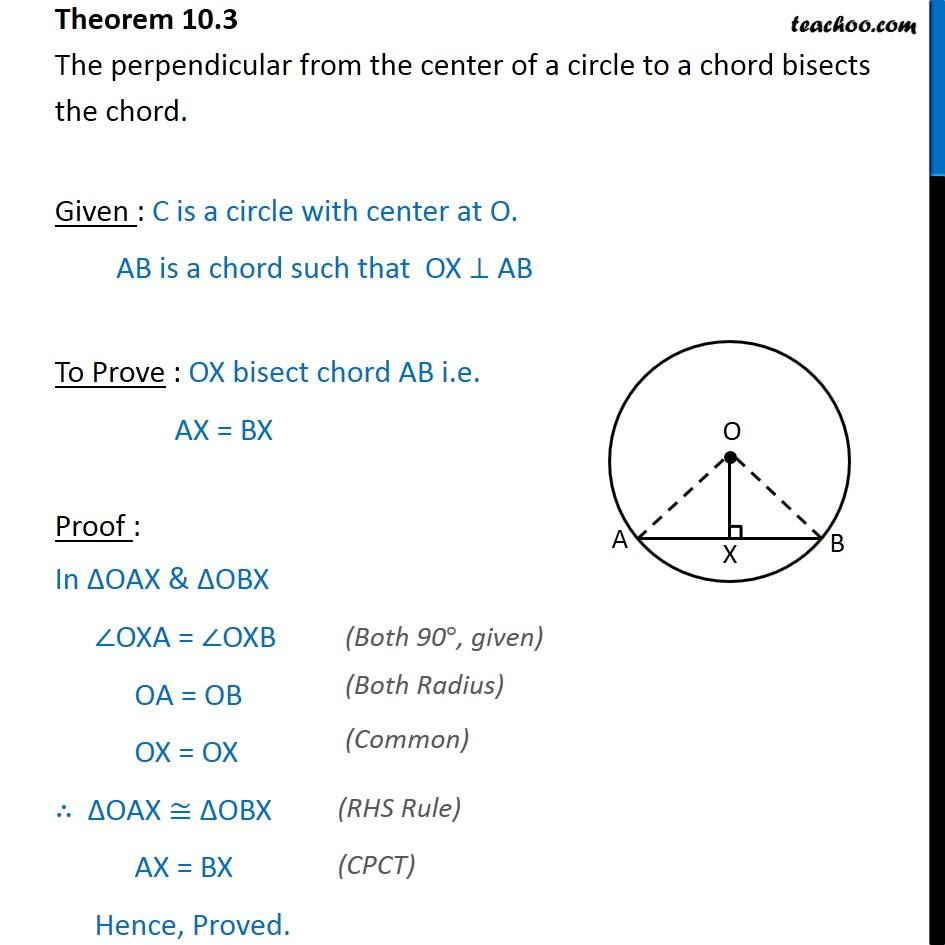1. Chapter 10 Class 9 Circles (Term 2)
2. Serial order wise
3. Theorems

Transcript

Theorem 10.3 The perpendicular from the center of a circle to a chord bisects the chord. Given : C is a circle with center at O. AB is a chord such that OX ⊥ AB To Prove : OX bisect chord AB i.e. AX = BX Proof : In ∆OAX & ∆OBX ∠OXA = ∠OXB OA = OB OX = OX ∴ ∆OAX ≅ ∆OBX AX = BX Hence, Proved.